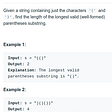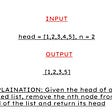# Understanding binary search: A super-simplified explanation

## Fundamentals and some examples in python

Searching for something from a data structure is a pretty common thing. We search for files, people, posts, etc. Most websites and applications have a search bar.

When it comes to problem-solving, searching for some element can be an important intermediary or actionable step. Here, we explore some fundamentals of searching algorithms.

• If you have an array of `n` elements, the simple way to find out if an element `x` exists in that array is to write a for loop.
• We visit each element of the array and check if it is equal to `x`. If true, we confirm the position of x in the array by returning its index.
• If the condition is never met in the loop, it implies that the element `x` doesn’t exist in the array.
• Since we are writing a for loop to traverse the entire array, the time taken to complete the search is a function of the array length, that is `n`. So, time complexity = O(n).

As with any programming question, we want to optimize this. How?

We have a special scenario in case the array in question is a sorted array. That’s where binary search comes into play. Let us see how we can take advantage of the structure of a sorted array to perform a more efficient search.

• We know that in a sorted array, all elements less than `x` will be to its left and those greater than `x` will be to the right.
• Let us assume the middle element of the array as `m`. Now, ask yourself a question. Where is `x` w.r.t `m`?
• Can we write like this?
`case 1: if x < m then x is to the left of middle.case 2: if x > m then x is to the right of middle.case 3: x = m and hence we can return the index of middle.`
• In case 1, x is somewhere in the subarray[0:m-1]. Can we repeat the same process again? Let’s find the middle element of this new subarray and compare it with `x`. By doing that we can determine the new sub-subarray where `x` is located in.
• The same thing can be done in case 2 as well. At each step, we find the middle element of the array and determine if x is to the left or right side of it. Then we isolate that new subarray and repeat the process until we get `x = middle element of that sub array`.
• This is the binary search algorithm. Since we break the size of our array into half at every step, we perform much lesser steps than when writing a for loop. The time complexity is O(log n).

Let’s do a dry run on a sample array to get the feel of how binary search is happening.

• Let’s say we have a sorted array = `[11, 12, 13, 14, 15, 16, 17, 18, 19, 20]`.
• Here’s the question- find the index of `19`. We do a binary search for the same.
• The first step is to find the middle elements. The length of the array = `10`. We can take `index = 10/2 = 5` for the middle element. In case the array size is odd, we can round off the value or take the int of `n/2`.
• `array = 16`.
• `19 > 16`. So `19` is to the right of `16`.
• The next step is to create the right side subarray, `[17, 18, 19, 20]`. To do this, we can keep track of the index of the first and last element of the array and then update that index accordingly.
• Let’s say `a = 0`, the first index and `b = 9`which is the last index. In the first step, we put `5` as the middle index. The right side subarray will start from index `6`. So, we can update the value of `a = 5 + 1 = 6`. `b = 9` still holds.
• We repeat the process again. We find the middle index for this new subarray, i.e `(6 + 9)/2 = 7.5 ~ 8`.
• `array = 19` which is the number we were searching for. So, we can now return its index = `8`.
• For a huge array, we repeat the same process until we find that the middle element of our new subarray is equal to the required element. If this condition is never met, it implies that the value is not present in the array.

Let us test our understanding further by solving a Leetcode problem. We will write code for the logic discussed above.

Write an efficient algorithm that searches for a value in an `m x n` matrix. This matrix has the following properties:

Integers in each row are sorted from left to right.

The first integer of each row is greater than the last integer of the previous row.

• In this question, we are given a list of lists and we have to find if the target number is present in that input matrix.
• For example, `matrix = [[1, 2, 3], [4, 5, 6], [7, 8, 9]]`. This can essentially be visualized into a 3*3 matrix. We have to return true if the target element exists in the matrix.
• The idea is once again exactly the same. Since you have a list of arrays with the condition such that all arrays are in ascending order, all you need to do is, visit each array and perform a binary search until you find the target element.

Here’s the python solution for the same. Do read the code comments and introspect on what’s happening.

Now that you have a fundamental understanding, try out this question on Leetcode by yourself. If possible, try to implement the solution in both python and javascript.

To test your understanding further you can try this problem too. You can solve it directly via binary search with some minor tweaks to the return statement.

Remember the fundamental search approach:

`1. Find out if a target element is to the left or right side of the middle element.2. Store the first and last index as variables and update them to create new subarrays where the target element might be present.3. Repeat this process till the middle element becomes equal to the target element.`

--

--

--

## More from The Research Nest

Empowering humanity with exclusive insights.

## Recommended from Medium## Publishing UPS metrics into the AWS CloudWatch## SenSwap — The Liquidity Accumulator## Leetcode 3. Longest Substring Without Repeating Characters## JACS — FAQS## How To Ensure The Reliability of Digital Services And IT Infrastructure And Reduce IT Management…## 32. Longest Valid Parentheses Leetcode Dynamic Programming## How We’ll Support You 24/7, Forever## XQ

Tech 👨‍💻 | Astronomy 🌠 | Life 🌱 | Poetry 🖊️ | Careers 👔

## LeetCode Patterns Adventure 24 — Maximum Depth of Binary Tree## Sorting Algorithms Explained Using Python: Insertion Sort## Remove nth node from end of list Leetcode Python## What is divide-and-conquer algorithm?